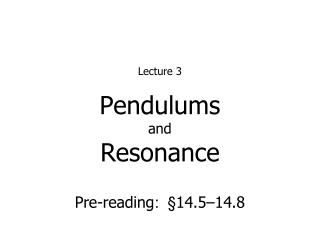DownloadDownload PresentationPendulums and Resonance

# Pendulums and Resonance

Download Presentation## Pendulums and Resonance

- - - - - - - - - - - - - - - - - - - - - - - - - - - E N D - - - - - - - - - - - - - - - - - - - - - - - - - - -
##### Presentation Transcript

1. PendulumsandResonance • Lecture 3 Pre-reading: §14.5–14.8

2. SHM: Pendulums • Simple pendulum: point mass on a massless, unstretched string • Exhibits SHM with ω = √(g/L)provided the amplitude (angle) is small (θ≲15°) • For real pendulum, need to know mass (m), distance to center of massd, and moment of inertiaI about rotation axisω = √(mgd/I)provided the amplitude (angle) is small (θ≲15°) §14.5–14.6

3. If friction force varies linearly with speed, we can solve for motion: with b describing the amount of damping Damped Oscillations • In real world, friction causes oscillations to decrease in amplitude §14.7

4. Critical dampingif b=2√(km) ⇒ ωʹ=0 returns to equilibrium without oscillating! • Underdampedb < 2√(km) • Overdampedb > 2√(km) Effects of damping • Amplitude decays exponentially. • Energy decays exponentially. • Angular frequency decreases.

5. Compare driving frequency with ‘natural frequency’ • If ωd ≃ωʹthen system oscillates with resonant behaviour: amplitude gets very large Forced Oscillations • Now we add a driving force to a damped harmonic oscillator • Suppose driving force is sinusoidal with driving frequency ωd §14.8

6. Mechanical waves • Read §15.1–15.2 Next lecture